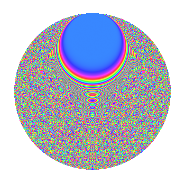# Properties

 Label 1089.2.vLevel 1089 Weight 2 Character orbit v Rep. character $$\chi_{1089}(37,\cdot)$$ Character field $$\Q(\zeta_{55})$$ Dimension 2160 Sturm bound 264

# Related objects

## Defining parameters

 Level: $$N$$ = $$1089 = 3^{2} \cdot 11^{2}$$ Weight: $$k$$ = $$2$$ Character orbit: $$[\chi]$$ = 1089.v (of order $$55$$ and degree $$40$$) Character conductor: $$\operatorname{cond}(\chi)$$ = $$121$$ Character field: $$\Q(\zeta_{55})$$ Sturm bound: $$264$$

## Dimensions

The following table gives the dimensions of various subspaces of $$M_{2}(1089, [\chi])$$.

Total New Old
Modular forms 5440 2240 3200
Cusp forms 5120 2160 2960
Eisenstein series 320 80 240

## Trace form

 $$2160q + 40q^{2} + 10q^{4} + 39q^{5} - 36q^{7} + 52q^{8} + O(q^{10})$$ $$2160q + 40q^{2} + 10q^{4} + 39q^{5} - 36q^{7} + 52q^{8} - 42q^{10} + 53q^{11} - 47q^{13} + 72q^{14} - 2q^{16} + 58q^{17} - 36q^{19} + 50q^{20} - 112q^{22} + 50q^{23} + q^{25} + 60q^{26} - 68q^{28} + 40q^{29} - 69q^{31} - 4q^{32} - 36q^{34} + 44q^{35} - 40q^{38} + 45q^{40} + 64q^{41} - 28q^{43} + 89q^{44} - 12q^{46} + 58q^{47} + 194q^{50} - 214q^{52} + 68q^{53} - 140q^{55} + 125q^{56} - 95q^{58} + 27q^{59} - 68q^{61} + 71q^{62} - 28q^{64} + 49q^{65} + 23q^{67} + 16q^{68} - 124q^{70} + 81q^{71} - 110q^{73} + 14q^{74} + 168q^{76} - 90q^{77} - 68q^{79} - 113q^{80} - 86q^{82} + 78q^{83} + 22q^{85} + 60q^{86} + 153q^{88} + 17q^{89} + 39q^{91} + 34q^{92} + 151q^{94} - 14q^{95} + 70q^{97} + 84q^{98} + O(q^{100})$$

## Decomposition of $$S_{2}^{\mathrm{new}}(1089, [\chi])$$ into newform subspaces

The newforms in this space have not yet been added to the LMFDB.

## Decomposition of $$S_{2}^{\mathrm{old}}(1089, [\chi])$$ into lower level spaces

$$S_{2}^{\mathrm{old}}(1089, [\chi]) \cong$$ $$S_{2}^{\mathrm{new}}(121, [\chi])$$$$^{\oplus 3}$$$$\oplus$$$$S_{2}^{\mathrm{new}}(363, [\chi])$$$$^{\oplus 2}$$

## Hecke Characteristic Polynomials

There are no characteristic polynomials of Hecke operators in the database